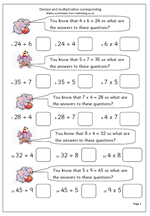# Free maths worksheet: Division and multiplication correspondingThis maths worksheet looks at the correspondence between multiplication and division. Given one fact, three others can be worked out. For example:

Given that  5 x 4 = 20 then:

a. 4 x 5 = 20

b. 20 ÷ 5 = 4

c. 20 ÷ 4 = 5

A full understanding of this relationship is needed before children can go on to written methods of division. If your child is struggling with division it would be well worth checking that they understand this.

To make sense of this it is a good idea to ‘talk through’ what these number sentences say.

If 5 lots of 4 are 20 then 4 lots of 5 will also be 20.

If 20 sweets are shared equally between 4 people they get 5 each.

If 20 sweets are shared equally between 5 people they get 4 each.

Multiplication and division corresponding